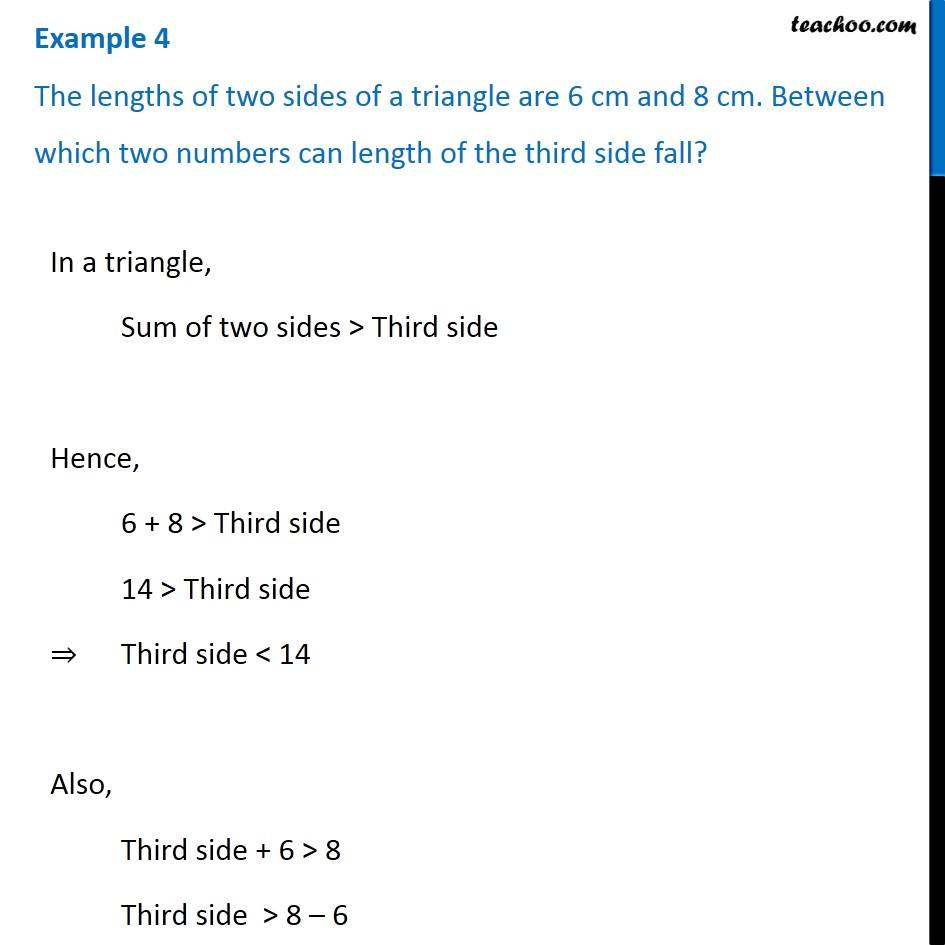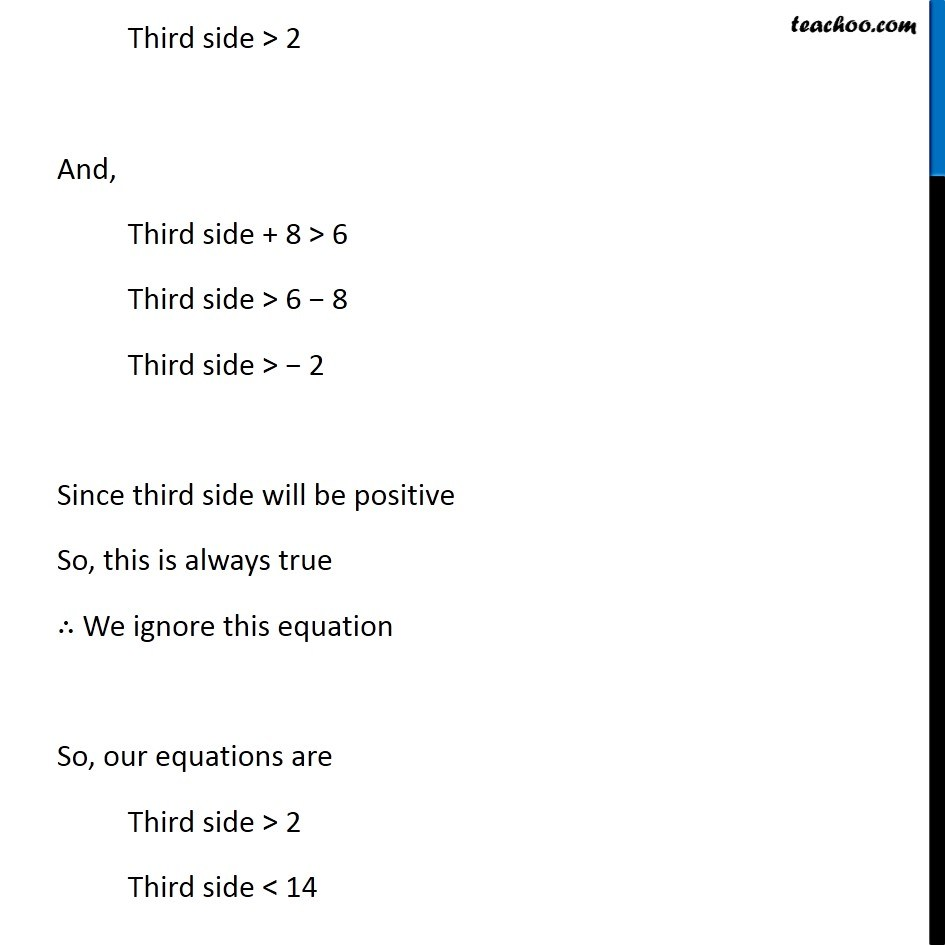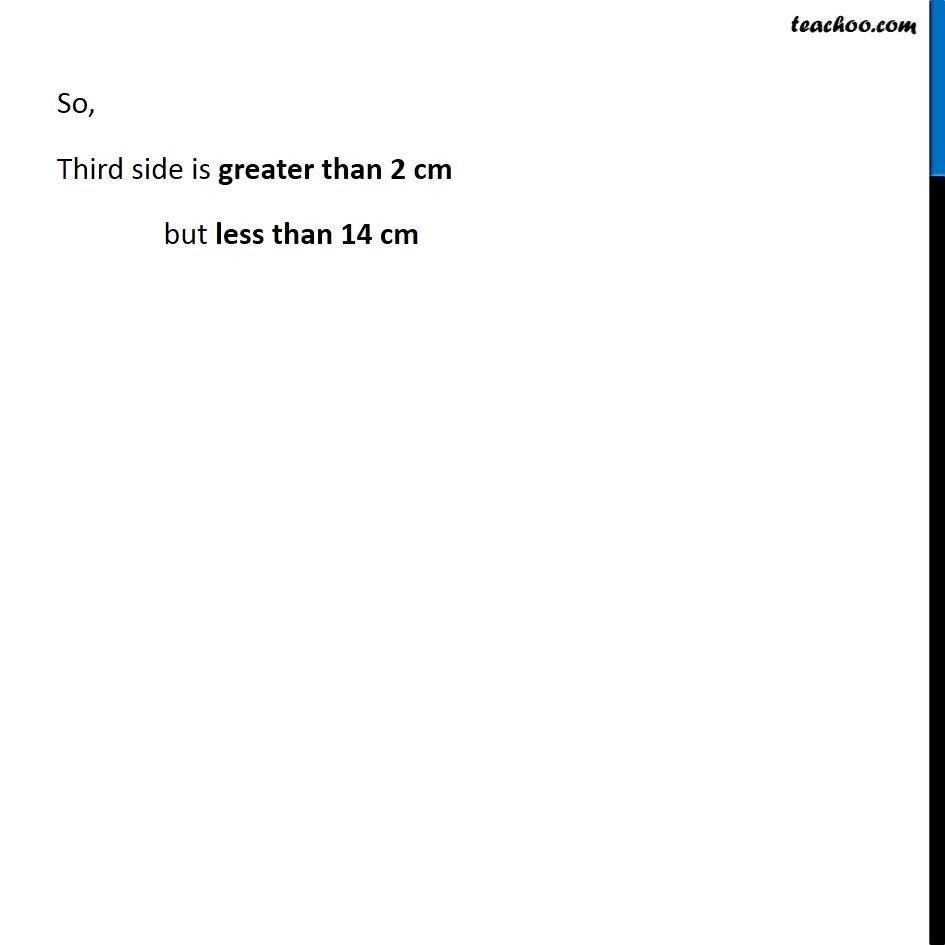Sum of lengths of two sides of a triangle

Chapter 6 Class 7 Triangle and its Properties
Concept wise### Transcript

Example 4 The lengths of two sides of a triangle are 6 cm and 8 cm. Between which two numbers can length of the third side fall? In a triangle, Sum of two sides > Third side Hence, 6 + 8 > Third side 14 > Third side ⇒ Third side < 14 Also, Third side + 6 > 8 Third side > 8 – 6 Third side > 2 And, Third side + 8 > 6 Third side > 6 − 8 Third side > − 2 Since third side will be positive So, this is always true ∴ We ignore this equation So, our equations are Third side > 2 Third side < 14 So, Third side is greater than 2 cm but less than 14 cm